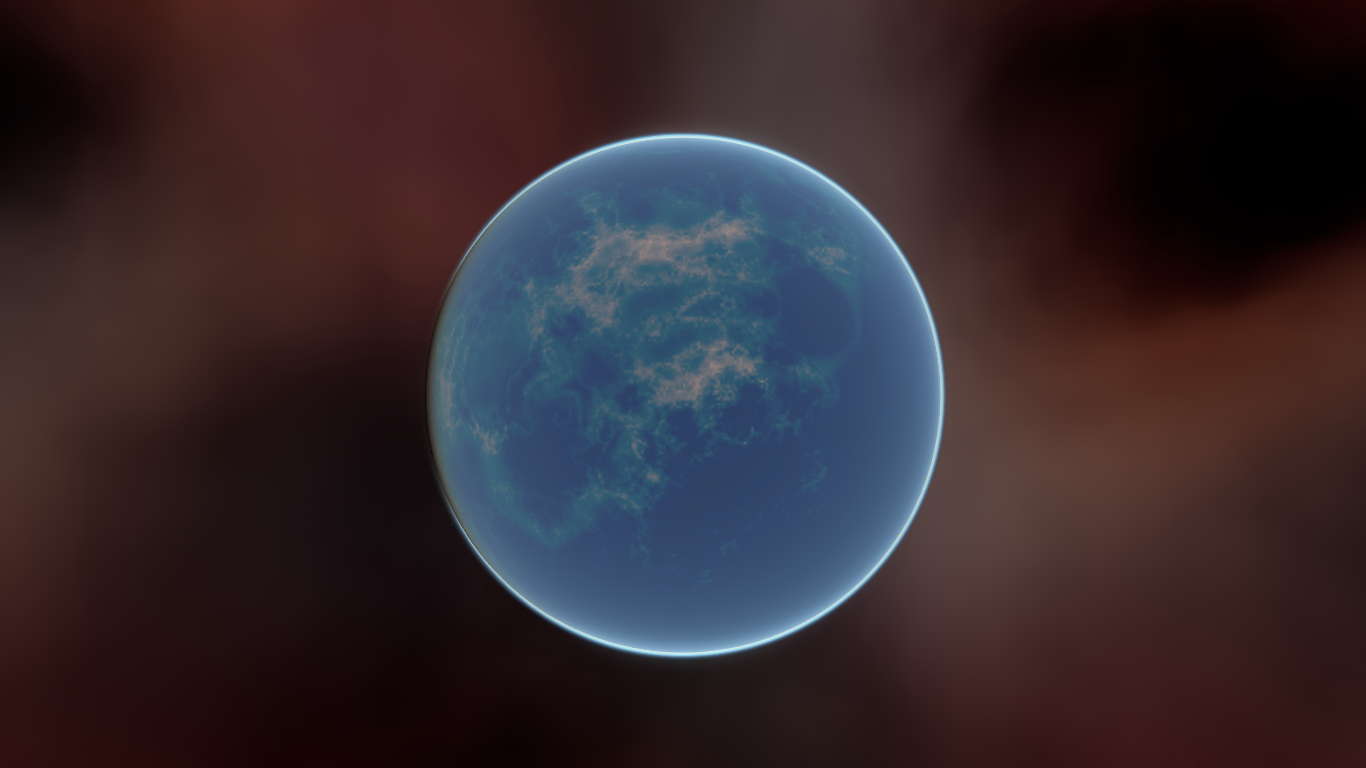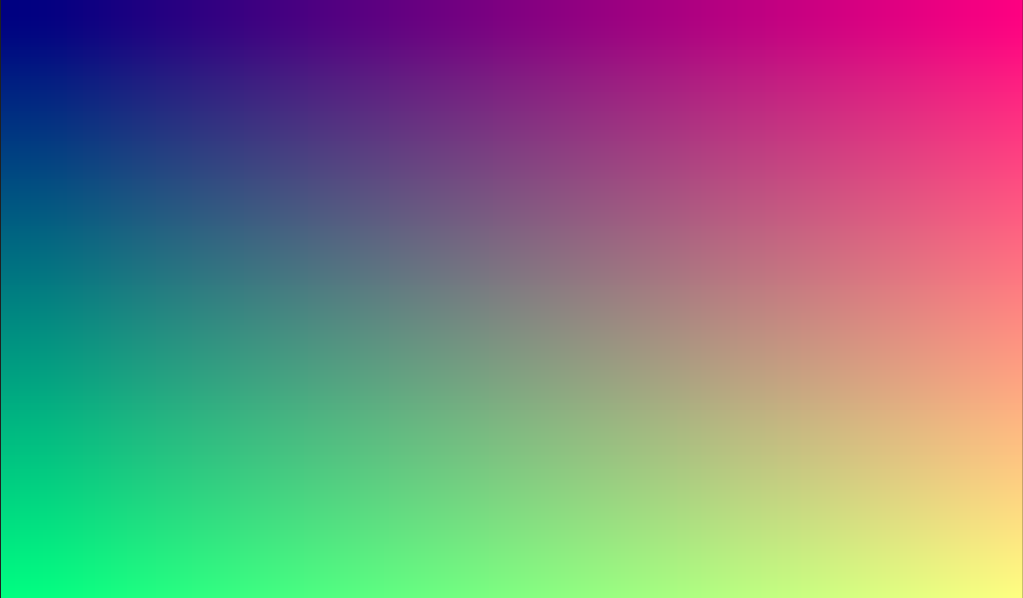# 使用视区作为纹理¶

## 简介¶

This tutorial will introduce you to using the Viewport as a texture that can be applied to 3D objects. In order to do so, it will walk you through the process of making a procedural planet like the one below:During the course of this tutorial, we’ll cover the following topics:

• 如何使用 Viewport 作为渲染纹理
• 使用equirectangular映射将纹理映射到球体
• 程序行星的碎片着色器技术
• 从a Viewport Texture 设置粗糙度贴图

## 设置视区¶

Next, set the size of the Viewport to `(1024, 512)`. The Viewport can actually be any size so long as the width is double the height. The width needs to be double the height so that the image will accurately map onto the sphere, as we will be using equirectangular projection, but more on that later.Next, disable HDR and disable 3D. We don’t need HDR because our planet’s surface will not be especially bright, so values between `0` and `1` will be fine. And we will be using a ColorRect to render the surface, so we don’t need 3D either.Next, we add a Shader Material to the ColorRect (ColorRect > CanvasItem > Material > Material > `New ShaderMaterial`).

Basic familiarity with shading is recommended for this tutorial. However, even if you are new to shaders, all the code will be provided, so you should have no problem following along.

```shader_type canvas_item;

void fragment() {
COLOR = vec4(UV.x, UV.y, 0.5, 1.0);
}
```## 应用纹理¶Then, from the menu that pops up, select the Viewport that we rendered to earlier.Your sphere should now be colored in with the colors we rendered to the Viewport.Notice the ugly seam that forms where the texture wraps around? This is because we are picking a color based on UV coordinates and UV coordinates do not wrap around the texture. This is a classic problem in 2D map projection. Game developers often have a 2-dimensional map they want to project onto a sphere, but when it wraps around, it has large seams. There is an elegant workaround for this problem that we will illustrate in the next section.

## 使行星纹理¶

So now, when we render to our Viewport, it appears magically on the sphere. But there is an ugly seam created by our texture coordinates. So how do we get a range of coordinates that wrap around the sphere in a nice way? One solution is to use a function that repeats on the domain of our texture. `sin` and `cos` are two such functions. Let’s apply them to the texture and see what happens.

```COLOR.xyz = vec3(sin(UV.x * 3.14159 * 4.0) * cos(UV.y * 3.14159 * 4.0) * 0.5 + 0.5);
```Not too bad. If you look around, you can see that the seam has now disappeared, but in its place, we have pinching at the poles. This pinching is due to the way Godot maps textures to spheres in its Spatial Material. It uses a projection technique called equirectangular projection, which translates a spherical map onto a 2D plane.

If you are interested in a little extra information on the technique, we will be converting from spherical coordinates into Cartesian coordinates. Spherical coordinates map the longitude and latitude of the sphere, while Cartesian coordinates are, for all intents and purposes, a vector from the center of the sphere to the point.

For each pixel, we will calculate its 3D position on the sphere. From that, we will use 3D noise to determine a color value. By calculating the noise in 3D, we solve the problem of the pinching at the poles. To understand why, picture the noise being calculated across the surface of the sphere instead of across the 2D plane. When you calculate across the surface of the sphere, you never hit an edge, and hence you never create a seam or a pinch point on the pole. The following code converts the `UVs` into Cartesian coordinates.

```float theta = UV.y * 3.14159;
float phi = UV.x * 3.14159 * 2.0;
vec3 unit = vec3(0.0, 0.0, 0.0);

unit.x = sin(phi) * sin(theta);
unit.y = cos(theta) * -1.0;
unit.z = cos(phi) * sin(theta);
unit = normalize(unit);
```

And if we use `unit` as an output `COLOR` value, we get:Now that we can calculate the 3D position of the surface of the sphere, we can use 3D noise to make the planet. We will be using this noise function directly from a Shadertoy:

```vec3 hash(vec3 p) {
p = vec3(dot(p, vec3(127.1, 311.7, 74.7)),
dot(p, vec3(269.5, 183.3, 246.1)),
dot(p, vec3(113.5, 271.9, 124.6)));

return -1.0 + 2.0 * fract(sin(p) * 43758.5453123);
}

float noise(vec3 p) {
vec3 i = floor(p);
vec3 f = fract(p);
vec3 u = f * f * (3.0 - 2.0 * f);

return mix(mix(mix(dot(hash(i + vec3(0.0, 0.0, 0.0)), f - vec3(0.0, 0.0, 0.0)),
dot(hash(i + vec3(1.0, 0.0, 0.0)), f - vec3(1.0, 0.0, 0.0)), u.x),
mix(dot(hash(i + vec3(0.0, 1.0, 0.0)), f - vec3(0.0, 1.0, 0.0)),
dot(hash(i + vec3(1.0, 1.0, 0.0)), f - vec3(1.0, 1.0, 0.0)), u.x), u.y),
mix(mix(dot(hash(i + vec3(0.0, 0.0, 1.0)), f - vec3(0.0, 0.0, 1.0)),
dot(hash(i + vec3(1.0, 0.0, 1.0)), f - vec3(1.0, 0.0, 1.0)), u.x),
mix(dot(hash(i + vec3(0.0, 1.0, 1.0)), f - vec3(0.0, 1.0, 1.0)),
dot(hash(i + vec3(1.0, 1.0, 1.0)), f - vec3(1.0, 1.0, 1.0)), u.x), u.y), u.z );
}
```

All credit goes to the author, Inigo Quilez. It is published under the `MIT` licence.

```float n = noise(unit * 5.0);
COLOR.xyz = vec3(n * 0.5 + 0.5);
```You can see now that the noise indeed wraps seamlessly around the sphere. Although this looks nothing like the planet you were promised. So let’s move onto something more colorful.

## 着色这个星球¶

Now to make the planet colors. While there are many ways to do this, for now, we will stick with a gradient between water and land.

To make a gradient in GLSL, we use the `mix` function. `mix` takes two values to interpolate between and a third argument to choose how much to interpolate between them; in essence, it mixes the two values together. In other APIs, this function is often called `lerp`. However, `lerp` is typically reserved for mixing two floats together; `mix` can take any values whether it be floats or vector types.

```COLOR.xyz = mix(vec3(0.05, 0.3, 0.5), vec3(0.9, 0.4, 0.1), n * 0.5 + 0.5);
```

The first color is blue for the ocean. The second color is a kind of reddish color (because all alien planets need red terrain). And finally, they are mixed together by `n * 0.5 + 0.5`. `n` smoothly varies between `-1` and `1`. So we map it into the `0-1` range that `mix` expects. Now you can see that the colors change between blue and red.That is a little more blurry than we want. Planets typically have a relatively clear separation between land and sea. In order to do that, we will change the last term to `smoothstep(-0.1, 0.0, n)`. And thus the whole line becomes:

```COLOR.xyz = mix(vec3(0.05, 0.3, 0.5), vec3(0.9, 0.4, 0.1), smoothstep(-0.1, 0.0, n));
```

What `smoothstep` does is return `0` if the third argument is below the first and `1` if the third argument is larger than the second and smoothly blends between `0` and `1` if the third number is between the first and the second. So in this line, `smoothstep` returns `0` whenever `n` is less than `-0.1` and it returns `1` whenever `n` is above `0`.One more thing to make this a little more planet-y. The land shouldn’t be so blobby; let’s make the edges a little rougher. A trick that is often used in shaders to make rough looking terrain with noise is to layer levels of noise over one another at various frequencies. We use one layer to make the overall blobby structure of the continents. Then another layer breaks up the edges a bit, and then another, and so on. What we will do is calculate `n` with four lines of shader code instead of just one. `n` becomes:

```float n = noise(unit * 5.0) * 0.5;
n += noise(unit * 10.0) * 0.25;
n += noise(unit * 20.0) * 0.125;
n += noise(unit * 40.0) * 0.0625;
```And with shading turned back on, it looks like:## 制作海洋¶

```COLOR.a = 0.3 + 0.7 * smoothstep(-0.1, 0.0, n);
```

This line returns `0.3` for water and `1.0` for land. This means that the land is going to be quite rough, while the water will be quite smooth.

And then, in the material, under the “Metallic” section, make sure `Metallic` is set to `0` and `Specular` is set to `1`. The reason for this is the water reflects light really well, but isn’t metallic. These values are not physically accurate, but they are good enough for this demo.

Next, under the “Roughness” section, set `Roughness` to `1` and set the roughness texture to a Viewport Texture pointing to our planet texture Viewport. Finally, set the `Texture Channel` to `Alpha`. This instructs the renderer to use the `alpha` channel of our output `COLOR` as the `Roughness` value.You’ll notice that very little changes except that the planet is no longer reflecting the sky. This is happening because, by default, when something is rendered with an alpha value, it gets drawn as a transparent object over the background. And since the default background of the Viewport is opaque, the `alpha` channel of the Viewport Texture is `1`, resulting in the planet texture being drawn with slightly fainter colors and a `Roughness` value of `1` everywhere. To correct this, we go into the Viewport and set “Transparent Bg” to on. Since we are now rendering one transparent object on top of another, we want to enable `blend_premul_alpha`:

```render_mode blend_premul_alpha;
```

This pre-multiplies the colors by the `alpha` value and then blends them correctly together. Typically, when blending one transparent color on top of another, even if the background has an `alpha` of `0` (as it does in this case), you end up with weird color bleed issues. Setting `blend_premul_alpha` fixes that.